Next: Real-Time Implementation Up: Real-Time Symmetry Transform Previous: Lines of Symmetry

## Intersection of Lines of Symmetry

Sela proposes that the intersection of the lines of symmetry in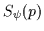generates an interest point. This interest point has a magnitude depending on the configuration and strength of the lines of symmetry that generated it. We utilize the magnitude of the interest point as a measure of the level of symmetric enclosure at that point. Since perpendicular lines of symmetry generate the strongest sense of enclosure , the greater the level of orthogonality between two lines of symmetry, the stronger their contribution to the interest magnitude at point p. The contribution of each pair of lines of symmetry intersecting point p is summed to generate an interest value I(p) which is defined as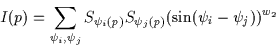(2.5)

where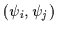are the orientation values of a pair of symmetry lines intersecting point p whose symmetry magnitudes are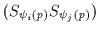. The effect of orthogonality is included in the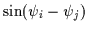term which is maximal when the lines of symmetry are perpendicular. The weight w2 is used to tune the sensitivity of the computation to the orthogonality of the intersecting lines of symmetry. A value of w2=5 is typically used so that orthogonal lines of symmetry dominate the response of the interest operator.Next: Real-Time Implementation Up: Real-Time Symmetry Transform Previous: Lines of Symmetry
Tony Jebara
2000-06-23